# Construction Stage Analysis

Solution

## 1. What is Construction Stage Analysis?

During construction, the bridge undergoes changes in its structural system, boundary conditions, material properties over time, and changes in the order and time in which the load acts. These changes must be reviewed because they generate different displacement and member forces at each construction stage, and may act more critical on the structure than the service stage.

•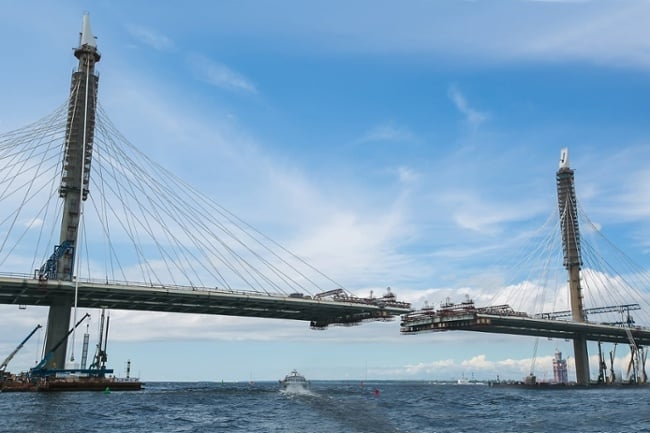Fig. Construction stage analysis

Although there are differences depending on the bridge type and construction method, this phenomenon mainly occurs due to the time-dependent material properties, deformation of tendons or cables, and prestress loss. The change according to the time-dependent material properties is a phenomenon that occurs in all bridges using concrete regardless of the bridge type, and it is different from steel bridges.

Changes in the load, boundary conditions and material properties (creep, shrinkage, and changes in elastic modulus over time) of concrete during the construction stage must be applied in the analysis. Furthermore, the applied stress of tendons depends on the profile and prestressing forces of tendons. The tendon profile (defined as the distance from the neutral axis of the member to the tendon) should be considered when the neutral axis varies as a composite section at each construction stage. Prestressing forces should be considered for both the initial losses, such as friction or loss of anchorage, and losses that occur as the construction stage progresses, such as relaxation.

In the case of cable bridges such as cable-stayed bridges and suspension bridges, accurately evaluating the cable tension force is one of the most important parts in design and construction, and can be planned in advanced through the construction stage analysis. The initial tension force or length of the cable can be determined by backward analysis. The effect of member forces occurring in each construction stage and the effect of construction equipment such as derrick cranes can be reviewed by forward analysis, which analyzes in the order of construction stages.

Possible complications can be prevented in advance by controlling additional displacements or member forces that may occur during construction through the construction stage analysis, and the fabrication camber and camber in construction can be calculated in advance through the deflection calculation in order to increase constructability.

•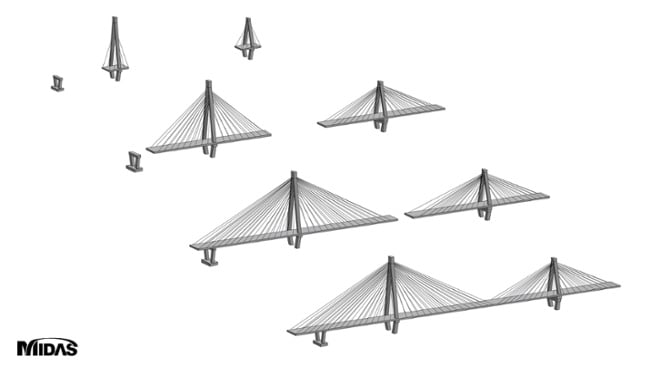Fig. Sequence of construction stage analysis

## 2. Time-Dependent Material Properties

As time passes, volume changes due to creep and shrinkage occur in concrete. These changes create stresses, cracks, and deflections, thus affecting the serviceability of the structure.

### A. Creep

#### A-1. Definition of Creep

As shown in the figure below, when a constant load is applied to concrete, initial instantaneous deformation occurs, and even though no additional load is applied, creep occurs, which increases the deformation over time. Creep deformation occurs quickly in the beginning, but the rate of increase gradually decreases over time. When the load is removed, elastic recovery and creep recovery occur, but permanent deformation remains.

•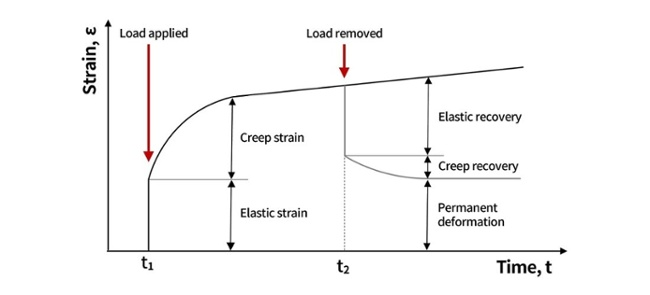Factors affecting concrete include the strength and age of concrete under load, sustained stress, duration of the load, relative humidity, temperature, size of the structure, water-cement ratio, and curing conditions.

One of the most important factors affecting creep externally is the relative humidity of the atmosphere surrounding concrete. In environments with low relative humidity, drying causes additional drying creep, which distinguishes from the basic creep term that occurs when drying is prevented. In general, creep refers to a phenomenon that combines the two cases.

If the magnitude of the applied sustained stress is within the elastic range of the stress-strain curve, the increase in creep strain maintains the same ratio. When the magnitude of the sustained stress exceeds the limit of proportionality (the limit in which the creep is proportional to the magnitude of the sustained stress), creep rate increases as the magnitude of the stress increases. If the strength is different due to the difference in age of the concrete, the creep strain is small when strength is high. Furthermore, creep recovery is also more likely to occur in late-age and dry concrete than in early-age concrete.

#### A-2. Creep Coefficient

The creep coefficient and specific creep used to calculate the deformation caused by creep are. If the applied stress is constant, simply multiply the stress value and the corresponding specific creep to obtain the creep strain. However, in most cases, stress continues to change according to the changes in external and internal loading conditions. In order to consider the time history of these stresses, it is assumed that the stresses are constant at short time intervals. Moreover, after obtaining each creep strain for the stress, linear superposition is performed to calculate the total creep strain.

The concept of creep coefficient, specific creep, and effective modulus of elasticity can be seen in the figure below. The effective modulus of elasticity can be used to consider the effects of creep and shrinkage when a long-term load is applied.

•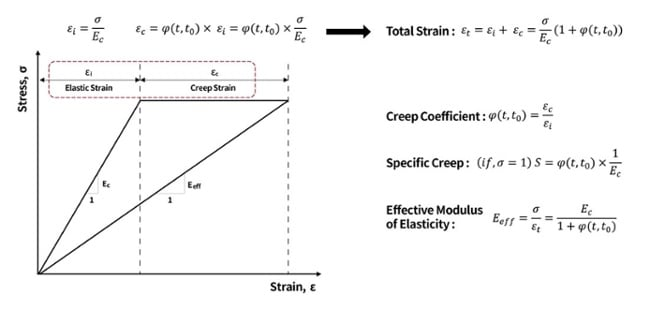Fig. Stress-strain curve due to sustained load

#### A-3. Calculation of Creep Coefficient According to Eurocode2

In the design standard, the creep coefficient is calculated and provided so that it can be applied in the design. In Annex B of the Eurocode 2 part 1-1, the process of calculating the creep coefficient is shown. The creep coefficient consists of the notional creep coefficient and a function that indicates how creep develops as a load begins to be loaded. In here, the notional creep coefficient consists of a function that represents the relative humidity, member thickness, concrete strength, and the effect of the duration of continuous load on creep. The development of creep over time can be calculated as a coefficient according to the relative humidity and member thickness, and as a correction factor according to the curing temperature and cement type. The overall formula for calculating the creep coefficient is shown in the figure below.

•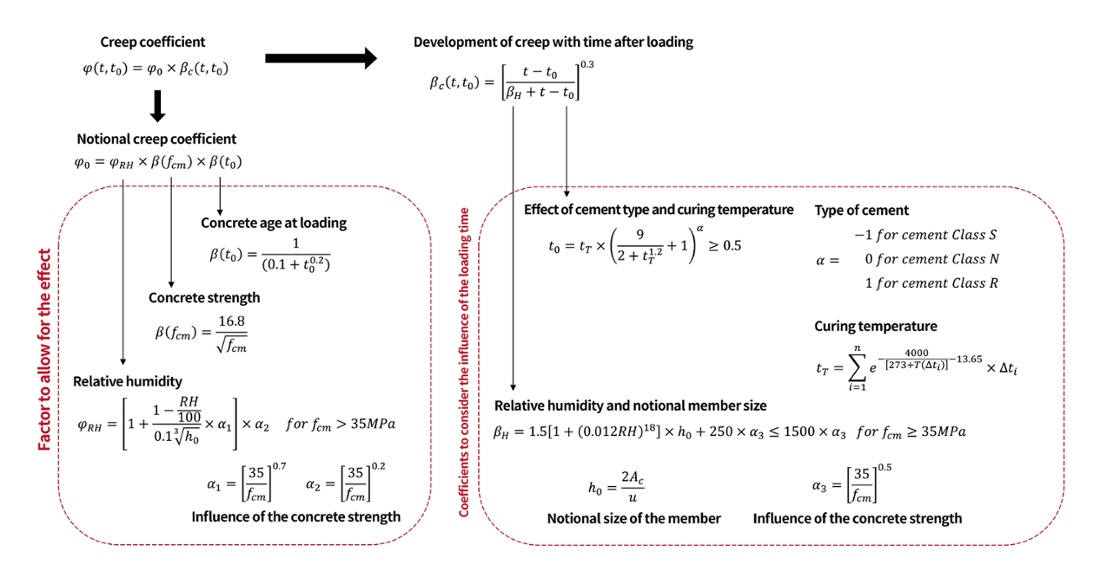Fig. Calculation of creep coefficient according to Eurocode2

The creep coefficient for a 1.0m x 1.0m square section was calculated using the formula provided by the Eurocode. The information required for calculating the concrete strength and relative humidity is assumed as follows. The structural analysis program provides creep coefficients according to each design standard, but it is highly recommended to check with manual calculations at least once.

•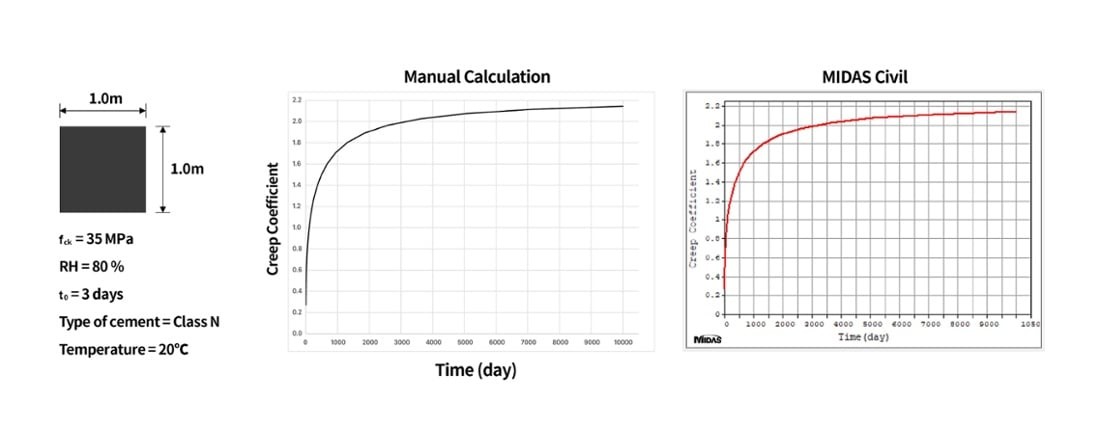Fig. Creep coefficient (manual calculation)

### B. Shrinkage

#### B-1. Definition of Shrinkage

When curing and drying of concrete starts, water not used for hydration moves from the inside of the concrete to the surface and evaporates. This phenomenon causes the concrete member to shrink over time, and the deformation caused by the reduction in volume due to the shrinkage is called drying shrinkage or shrinkage strain.

It is generally known that these same factors affect creep and shrinkage, and also occur simultaneously. There are several factors that affect shrinkage, and the most influencing factors are the unit content of water in concrete and relative humidity. Furthermore, when concrete is reinforced, shrinkage strain is reduced compared to members that are not, and moisture evaporation occurs more rapidly as the surface area exposed to the atmosphere is wider.

Water that’s close to the surface of the concrete evaporates rapidly, while water inside the concrete takes time to move to the surface, making the drying progress relatively slowly. Therefore, the initial drying shrinkage strain becomes larger near the surface than inside, causing compressive forces on the inside of concrete and tensile forces on the surface to maintain balance by itself. However, shear stresses occur because the deformation of the interface between the surface and inside of concrete must be the same. In addition to the internal factors, stress due to shrinkage occurs even when the concrete member is constrained in the direction of shrinkage.

#### B-2. Calculation of Shrinkage Strain According to Eurocode2

Similar to the calculation of the creep coefficient, the shrinkage strain can be applied in the design by the equation suggested by each design standard. In Eurocode 2 part 1-1, total shrinkage strain and autogenous shrinkage strain are given in Section 3.1.4, and equations and explanations for drying shrinkage strain are given in Annex B. The calculation formula is as follows:

•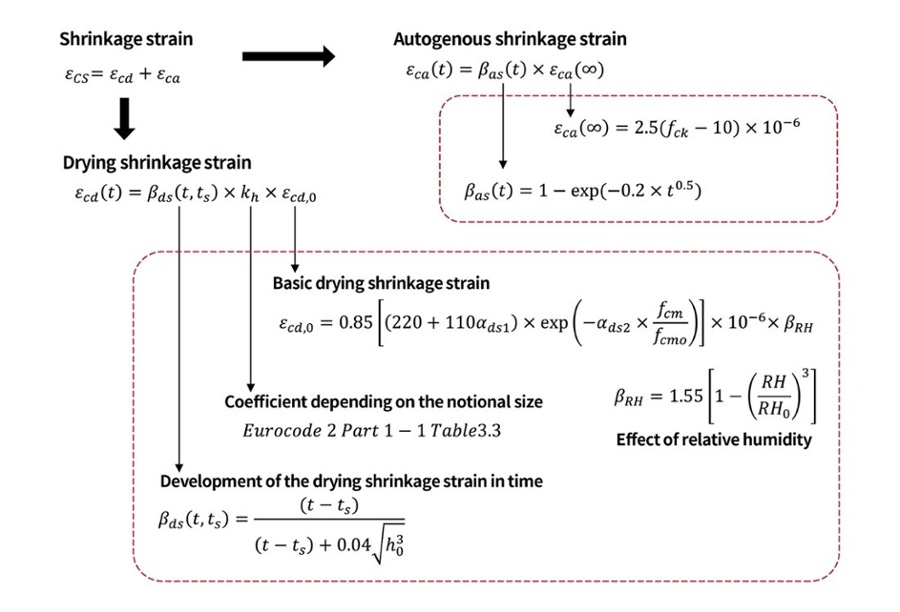Fig. Calculation of shrinkage strain according to Eurocode2

The figure below shows how the shrinkage strain is calculated manually using the information used to calculate the creep coefficient. Furthermore, these results can also be checked with the results obtained through the structural analysis program.

•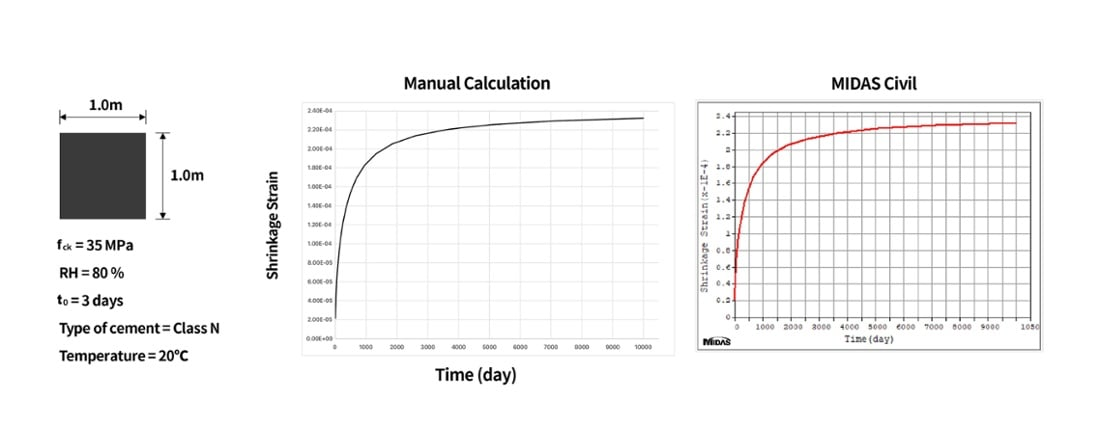Fig. Shrinkage strain (manual calculation)

### C. Changes in Modulus of Elasticity over Time

Since the compressive strength and modulus of elasticity of concrete varies with time, the specific strength of the concrete structure is not exhibited until a certain amount of time has passed. In concrete structures, including bridges, it is essential to reasonably consider this aging effect in order to predict the strength of concrete by construction stage analysis and apply it in the design.

## 3. Tendon Losses

The jacking force acting on the prestressed concrete fails to transmit all the forces to the concrete due to the instantaneous loss and the time dependent loss that occurs during the construction stage. Additionally, the neutral axis changes as the cross-section changes due to concrete placement according to the construction stage, and as the distance between the tendon and the neutral axis of the cross-section changes, the stress value applied to the member varies. For this reason, it is necessary to reflect the tendon force at each stage in the design through the construction stage analysis.

•Fig. Concept diagram of jacking force change due to loss

Transfer of prestress refers to tensioning of a prestressing tendon, which causes compressive forces to act on the concrete as a reaction to the jacking force. As shown in the figure above, this force, which is reduced due to the instantaneous loss, acts on the concrete as initial prestress. After the prestress is transferred into the concrete, the initial prestress decreases due to the time dependent loss. This reduced forced is called the effective prestress.

The most important factors in the design of prestressed concrete are the initial prestress and the effective prestress, and the relationship between the two forces can be expressed as the effectiveness ratio (‘R’). Generally, the effective prestress applied to the design is 80% of the initial prestress in the pre-tensioning method and 85% of the initial prestress in the post-tensioning method. The types and causes of loss, and differences according to the transfer of prestress can be summarized as follows:

### A. Loss Occurrence Order According to the Transfer of Prestress Method

#### A-1. Pre-tensioning Method

In the pre-tensioning method, the loss of prestress occurs at the jacking stage, the transfer of prestress stage, and the sustained loading stage. In the process of tensioning the tendons and anchored at the buttress, loss due to anchorage slip occurs and loss due to the initial relaxation begins. When prestress is transferred after the concrete hardens, loss due to elastic shortening occurs. When load is applied after completion of the construction, loss due to creep and shrinkage, and loss due to relaxation occur over time. Lastly, after time dependent loss occurs, the initial prestress state of the tendon becomes the effective prestress state.

•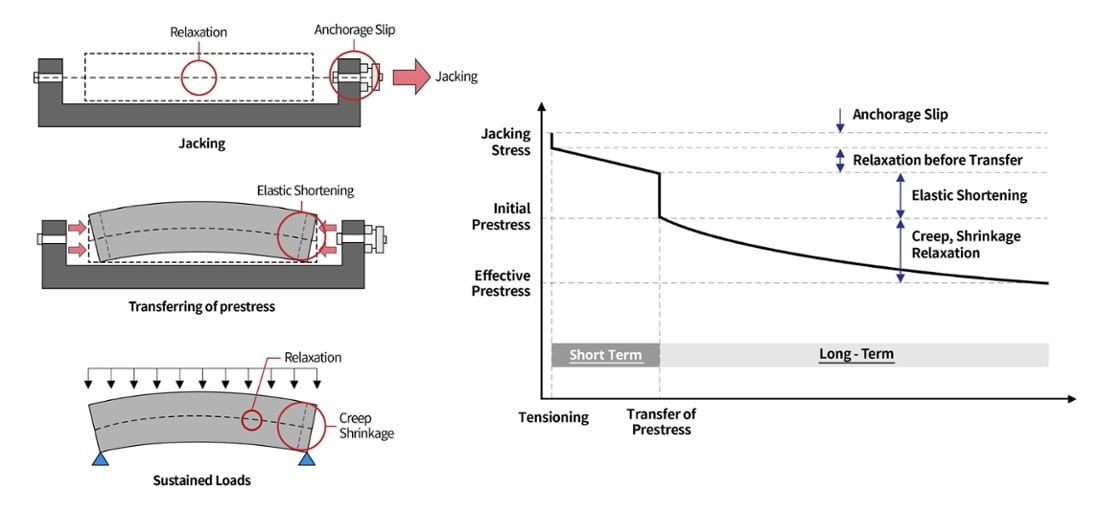Fig. Losses in pre-tensioning

#### A-2. Post-tensioning Method

In the post-tensioning method, the loss of prestress occurs at the transfer of prestress stage and the sustained loading stage. When the tendons are tensioned on the hardened concrete where the sheaths are arranged, loss due to elastic shortening and loss due to friction occur at the same time. Furthermore, when the prestressing jack is removed, the prestressing tendon settles, resulting in loss due to anchorage slip. These three losses occur almost simultaneously in the process of transfer of prestress. Additionally, just as in the pre-tensioning method, time dependent losses occur under the sustained load state, ultimately becoming an effective prestress state. The loss due to friction occurs only in the post-tensioning method using sheaths.

•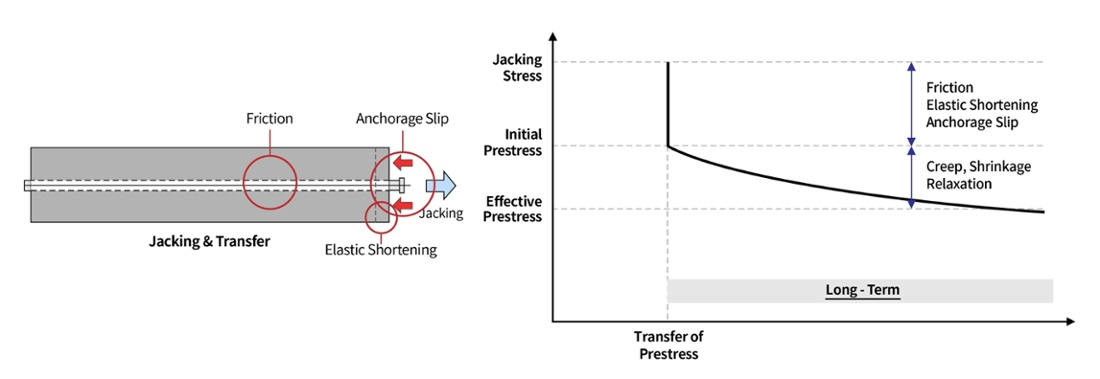Fig. Losses in post-tensioning

### B. Instantaneous Losses

The types and causes of instantaneous losses are as follows.

#### B-1. Loss due to Elastic Shortening

When prestress is transferred into concrete, the concrete is compressed and shortens due to elastic shortening. At this time the tendon also shortened, resulting in losses and which is called elastic shortening loss.

##### Elastic Shortening of Pre-tensioned Members

For pre-tensioned members, tendons are first tensioned and fixed onto a support. Once the tendons are settled, concrete is poured and cured. When prestress is transferred to the member after the concrete is hardened, elastic shortening occurs.

##### Elastic Shortening of Post-tensioned Members

For post-tension members, concrete is first poured and cured. Then, prestress is transferred by tensioning the tendons with a jack at this time elastic shortening occurs. For most post-tension members, tendons are tensioned and settled one-by-one in order instead of tensioning the tendons all at once. Therefore, the elastic shortening of concrete also occurs sequentially, and this must be applied in the design.

##### Sample Calculation of Prestress Loss due to Elastic Shortening

As an example, when two tendons are tensioned sequentially, tensioning the second tendon causes loss due to elastic shortening on the first tendon. The figure below shows the results of the final prestress of the first tendon after loss obtained through a structural analysis program. The losses due to friction, anchorage slip and relaxation were neglected.

•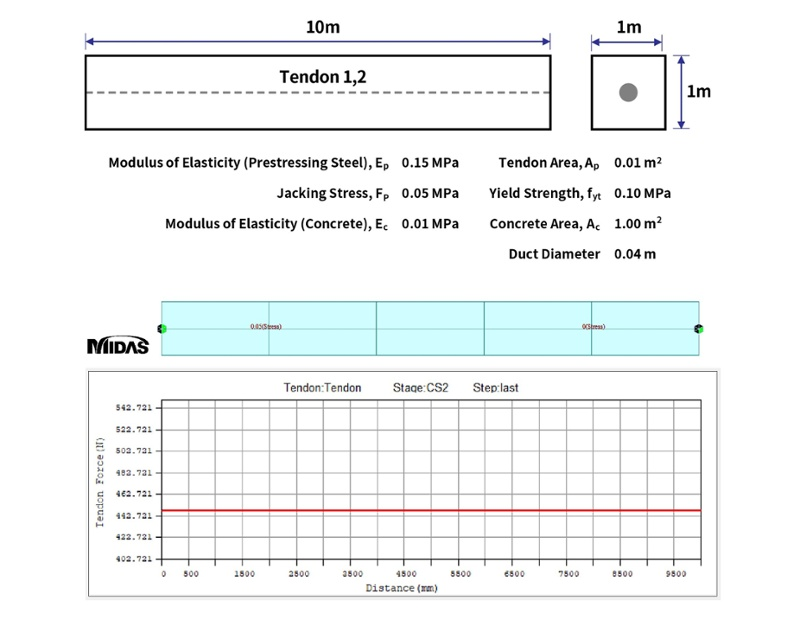Fig. Calculation of prestress loss due to elastic shortening

#### B-2. Loss due to Friction

The loss due to friction is a loss caused by frictional action that occurs when the stretched tendon contacts the sheaths during the post-tensioning method. The friction loss can be divided into the loss of curvature friction due to the curvature effect of the tendon and the loss of wobble friction due to the effect of the tendon’s length.

•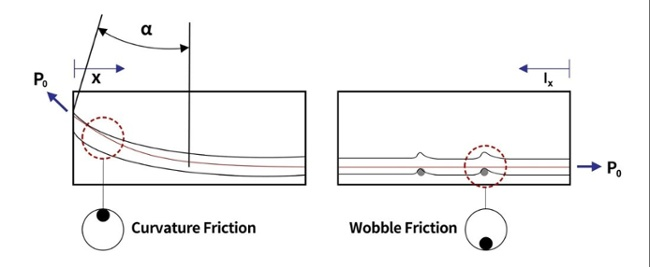Fig. Friction in post-tensioning system

##### Curvature Friction

Let’s assume curvature (‘α’, radian) at an arbitrary position separated by ‘x’ for a prestressing steel that is arranged in a curve and tensioned by a force P0. The prestress Px at this position can be expressed as the coefficient of curvature friction (‘μ’, 1/radian) and curvature (‘α’) as follows.

Px = P0 e - μα

The prestress P0 for the prestress Px after friction loss at position ‘x’ is as follows.

P0 = Px e^(μα)

##### Wobble Friction

Wobble friction refers to the occurrence of friction due to angular changes caused by local deformation of the sheath. The sheath is deflected by the influence of reinforcing bars or its own self-weight, and friction occurs when it comes into contact with the tendons. The prestress Px at a position separated by ‘x’ from the end where the prestress is transferred can be expressed by the length of the tendon (‘lx’, m) and the wobble friction (‘k’, 1/m).

Px = P0 e^(-klx)

Both curvature and wobble friction losses occur in post-tension members, and these two losses must be calculated by adding them together. The curvature friction coefficient and the wobble friction coefficient for the type of prestressing steal and the type of sheath are presented in the design standards.

Px = P0 e^{-(μα + klx)}

##### Sample Calculation of Prestress Loss due to Friction

The graph below can be obtained by calculating the prestress considering only frictional losses when a tendon is placed in a curve.

•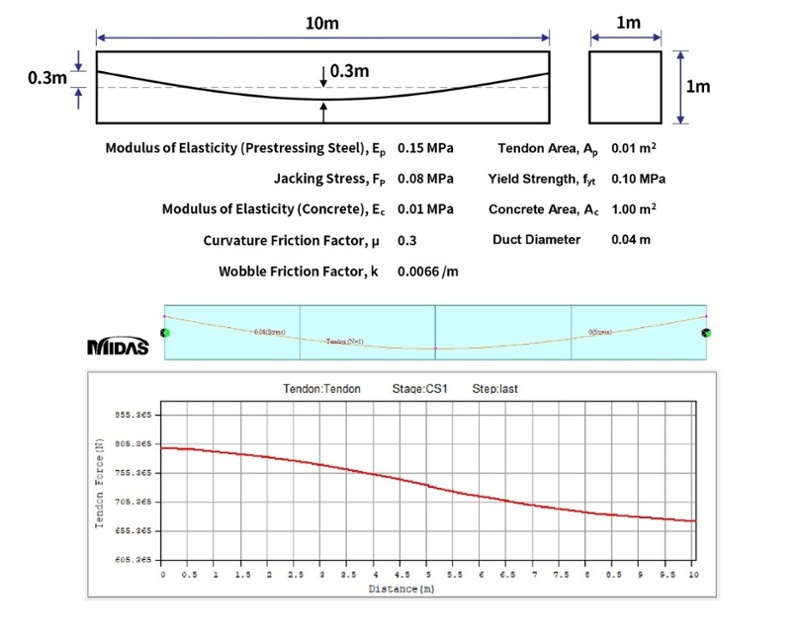Fig. Calculation of prestress loss due to friction of tendons arranged in a curve

#### B-3. Loss due to Anchorage Slip

As the tendon stretched by jacking is anchored to the anchorage, the anchorage system may be slightly pushed or moved. After this process, the tendon that had been stretched shortens and prestress is lost. The length of the anchorage slip varies depending on the type of anchorage, but loss is generally calculated by assuming anchorage slip at 6mm.

Loss due to anchorage slip is calculated by classifying the case with no friction (post-tension reduced friction using grease, pretension, etc) and the case with friction.

In the absence of friction, the loss of prestress due to the anchorage can be expressed as the elastic modulus (Ep) and cross-sectional area (AP) of the prestressing steel, the slip of anchorage (Δset), and the length between anchorages (lp).

•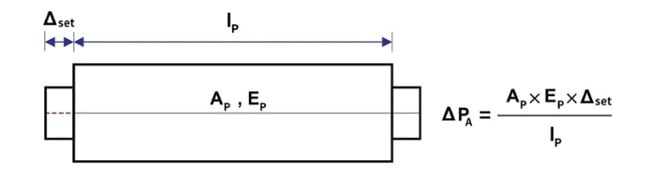Fig. Prestress loss due to anchorage slip in the absence of friction.

When friction is considered, the loss due anchorage is limited to the vicinity of the anchorage, and it does not have an effect when it moves away from the anchorage zone. The length of the section affected by the anchorage slip can be derived from the deformation analysis of the member subjected to the tensile force. Furthermore, the deformation analysis can be applied to the analysis of the anchorage loss of the post-tension member.

The length (lset) of the tendon, which is affected by the slip of the anchorage, is a function of friction loss: it shortens when the friction loss is large, and becomes longer when the friction loss is small.

•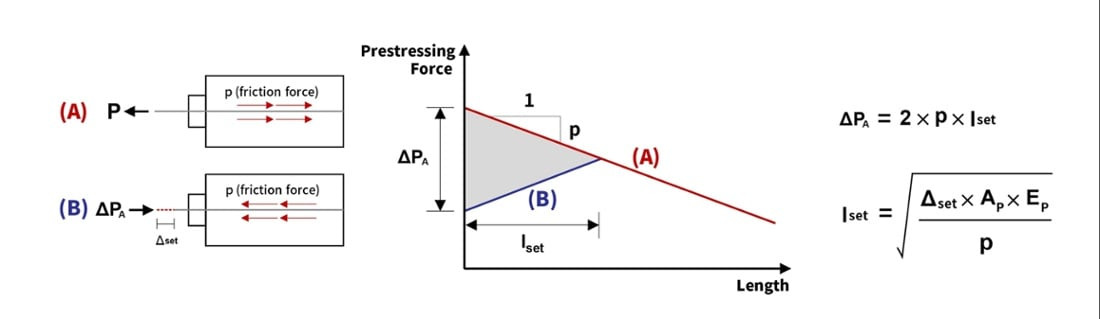Fig. Prestress loss due to anchorage slip in the presence of friction.

When tendon is tensioned, a frictional force is generated from the inside to the right, so the prestress force decreases as shown in (A). Removing the prestressing jack causes the tendon to settle in the anchorage, causing slip, and a frictional force to the left. This causes prestress loss. As distance from the anchorage zone increases, the frictional force decreases. Thus the loss is reduced like the graph (B).

##### Sample Calculation of Prestress Loss due to Anchorage Slip

In order to calculate the loss for anchorage slip in the presence of friction, additionally add 0.05m of anchorage slip to the sample calculation of friction loss in the above equation.

•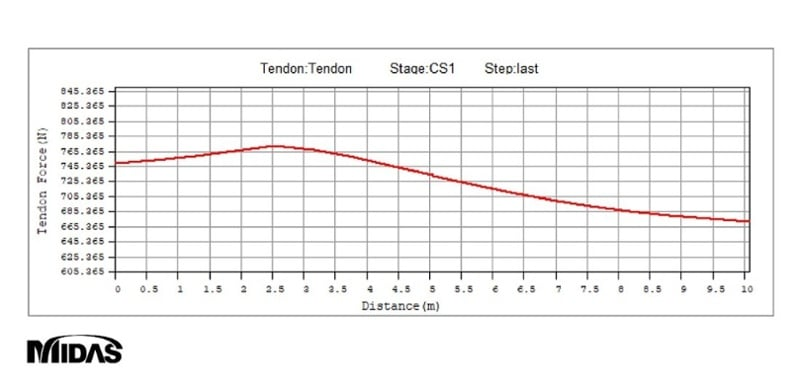Fig. Calculation of prestress loss due to anchorage slip in the presence of friction

### C. Time Dependent Losses

Types of time dependent losses include loss due to creep and shrinkage of concrete and relaxation of prestressing steel. Time dependent losses appear in both pre-tension and post-tension methods. The relaxation of prestressing steel interacts with the creep of concrete and is influenced by the shrinkage of concrete. Furthermore, it is difficult to accurately predict long-term losses because variability in material properties must be considered. Due to these problems, many design standards propose approximate solutions to estimate long-term losses by empirical research.

#### C-2. Loss due to Relaxation

A phenomenon in which tensile stress decreases over time when the prestressing steel is maintained at a constant length under tension. This phenomenon increases as the temperature increases and the stress increases. Therefore, for prestressing steel subjected to very high levels of tensile stress, the prestress decreases more due to relaxation.

Relaxation is divided into pure relaxation and apparent relaxation

##### Pure Relaxation

It is the amount of decrease in tensile stress that occurs when the strain is constant, and is expressed as a percentage of the initially applied tensile stress.

It is applied to the loss analysis by relaxation that occurs until the prestress is transferred after tensioning the prestressing tendon in the manufacture of pretension members.

##### Apparent Relaxation

After prestress is transferred, the relaxation behavior is changed due to the influence of concrete (creep, shrinkage), and the strain of tendon is not kept constant and decreases.

The relaxation that occurs when strain decreases over time is called apparent relaxation. Both pre-tension members and post-tension members should be reviewed.

##### Sample Calculation of Prestress Loss due to Relaxation

Under the same conditions as the sample calculations for the loss due to friction and anchorage slip, calculate the prestress considering the loss due to relaxation after 100 days. It was calculated using the equation proposed by ‘Magura’, with a relaxation coefficient (‘C’) of 45 (general steel C=10, low relaxation steel C=45).

•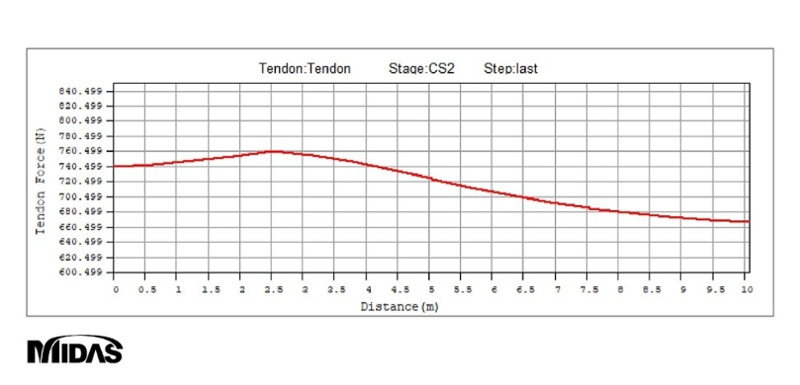Fig. Calculation of prestress loss due to relaxation after 100 days